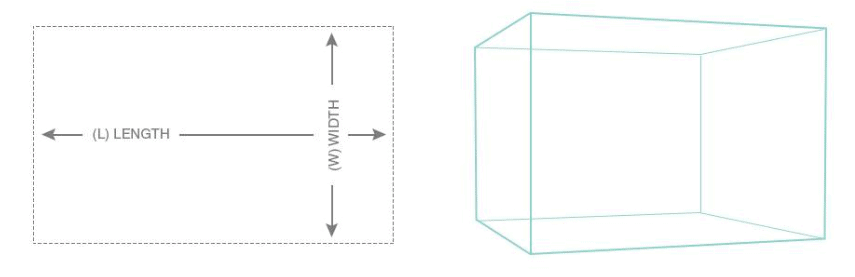# Aquarium Water Volume Calculator (Easy To Use)Calculating your aquarium water volume can be done easily. All you need to know is the dimensions of the tank. Once you know this, simply multiply them together and divide by 231.

Length x Width x Height  /231

You can also use this calculator online from Marine Depot.

If your aquarium is not rectangular in shape, calculating water volume becomes a bit more difficult. But using the online calculator makes it easy. Simply choose your tank shape and dimensions and the calculator does the rest.

Marine Depot water volume calculator here.